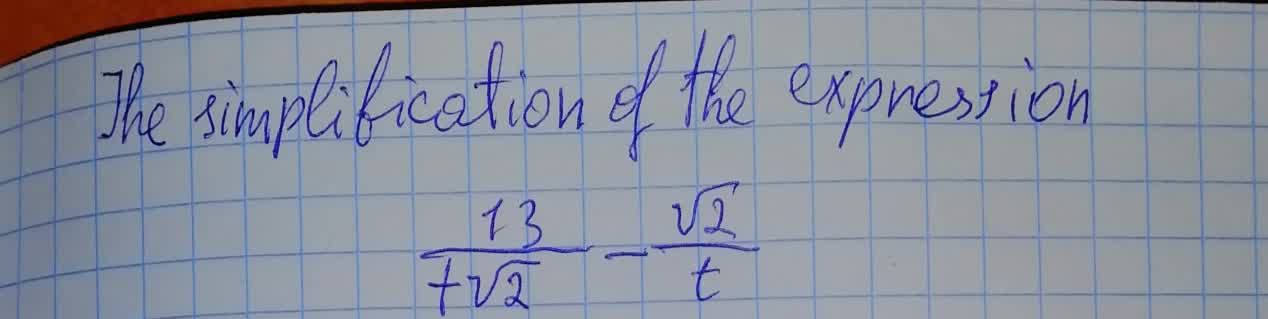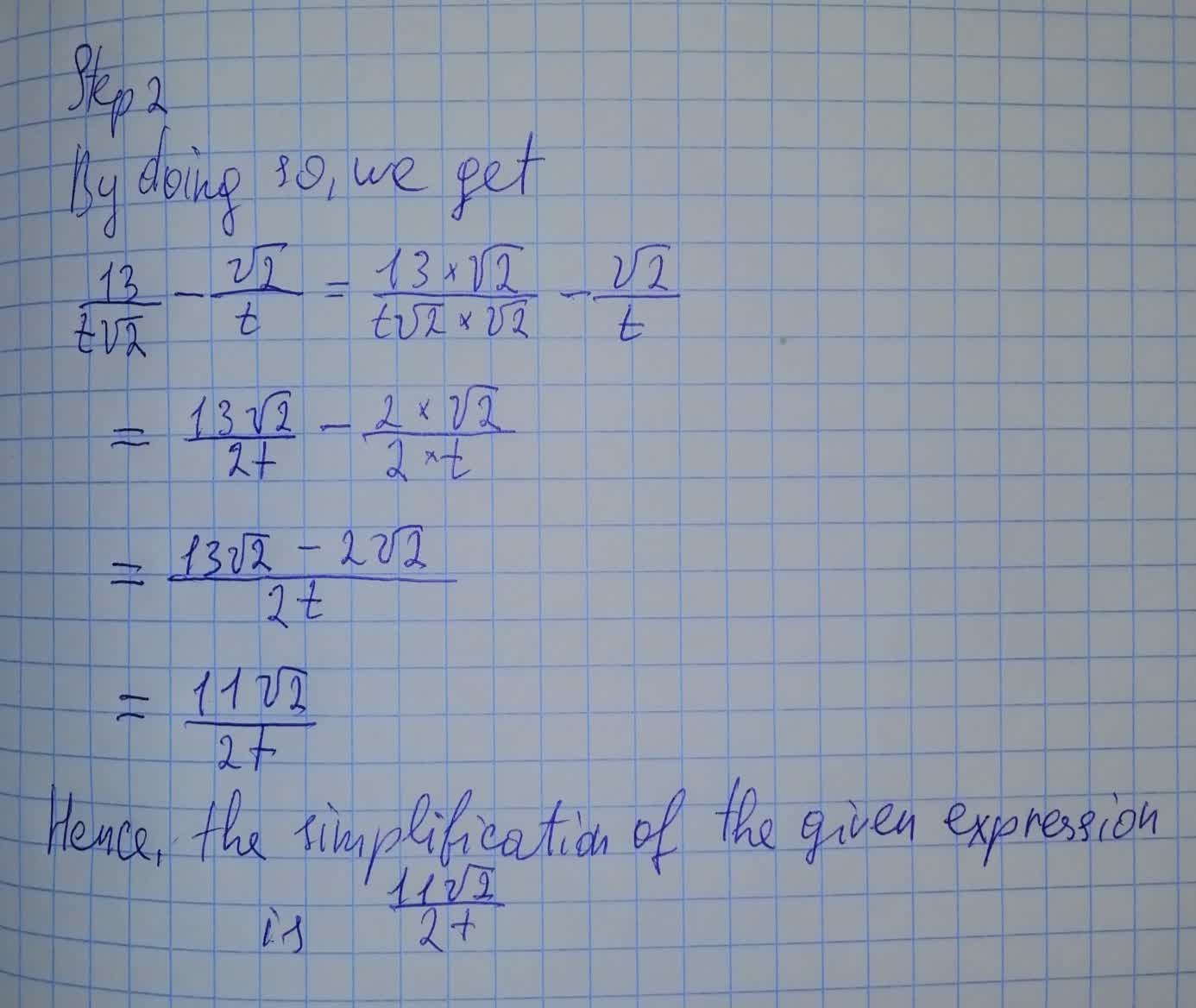Question# To calculate: The simplification of the expression \frac{13}{t\sqrt{2}}-\frac{\sqrt2}{t}

Conic sections
ANSWEREDTo calculate: The simplification of the expression $$\displaystyle{\frac{{{13}}}{{{t}\sqrt{{{2}}}}}}-{\frac{{\sqrt{{2}}}}{{{t}}}}$$